# Statistics, Science, Random Ramblings

## A blog mostly about data and R

I have been using R for over a decade now. Until I stumbled across `ggplot2` in a version pre 1.0 I thought that plots in R are kind of ugly and one of R’s weak points. Since then I my knowledge and skills regarding R have improved quite a bit, but I have never really bothered to have a second look at base plots.

Well, let’s change this.

We will be using the `palmerpenguins` data set.

``data("penguins", package = "palmerpenguins")``

``plot(penguins\$flipper_length_mm, penguins\$body_mass_g)``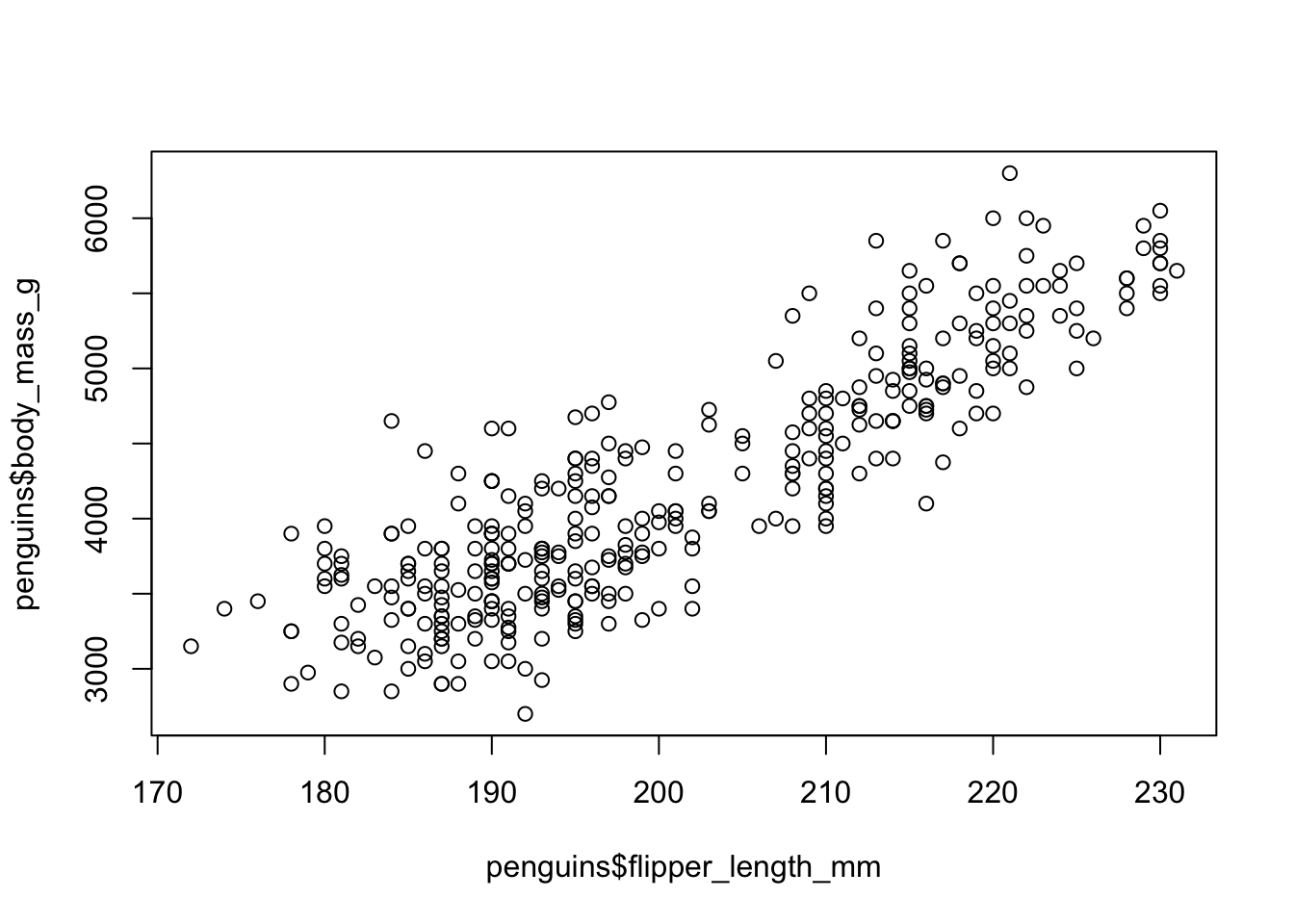This… does not look nice.

But we can do better! First, we can improve the axis labels and we also should be more specific when it comes to telling R which type of plot we want (the default is a scatter plot, but it is always good to be specific).

``````plot(x    = penguins\$flipper_length_mm,
y    = penguins\$body_mass_g,
type = "p",
xlab = "Flipper Length (mm)",
ylab = "Body Mass (g)")``````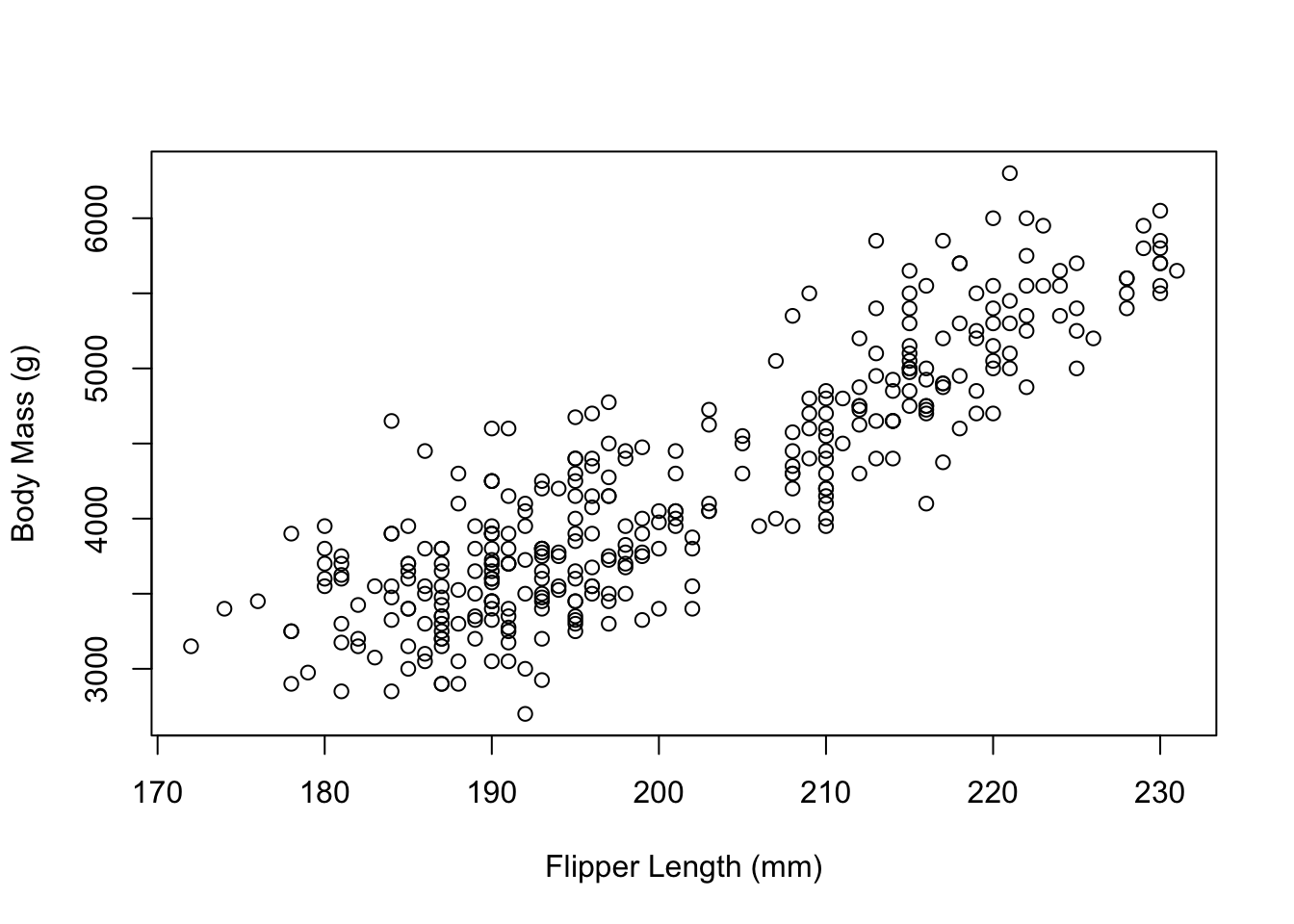Next, we might want to have a look at changing the box around the plot. By reading the documentation a bit, we soon find `graphics::par` giving us a list of graphical parameters we can set to change the appearance of our plot. Here, we find the `bty` parameter.

A character string which determined the type of box which is drawn about plots. If bty is one of “o” (the default), “l”, “7”, “c”, “u”, or “]” the resulting box resembles the corresponding upper case letter. A value of “n” suppresses the box.

We probably want `l`.

``````plot(x    = penguins\$flipper_length_mm,
y    = penguins\$body_mass_g,
type = "p",
xlab = "Flipper Length (mm)",
ylab = "Body Mass (g)",
bty  = "l")``````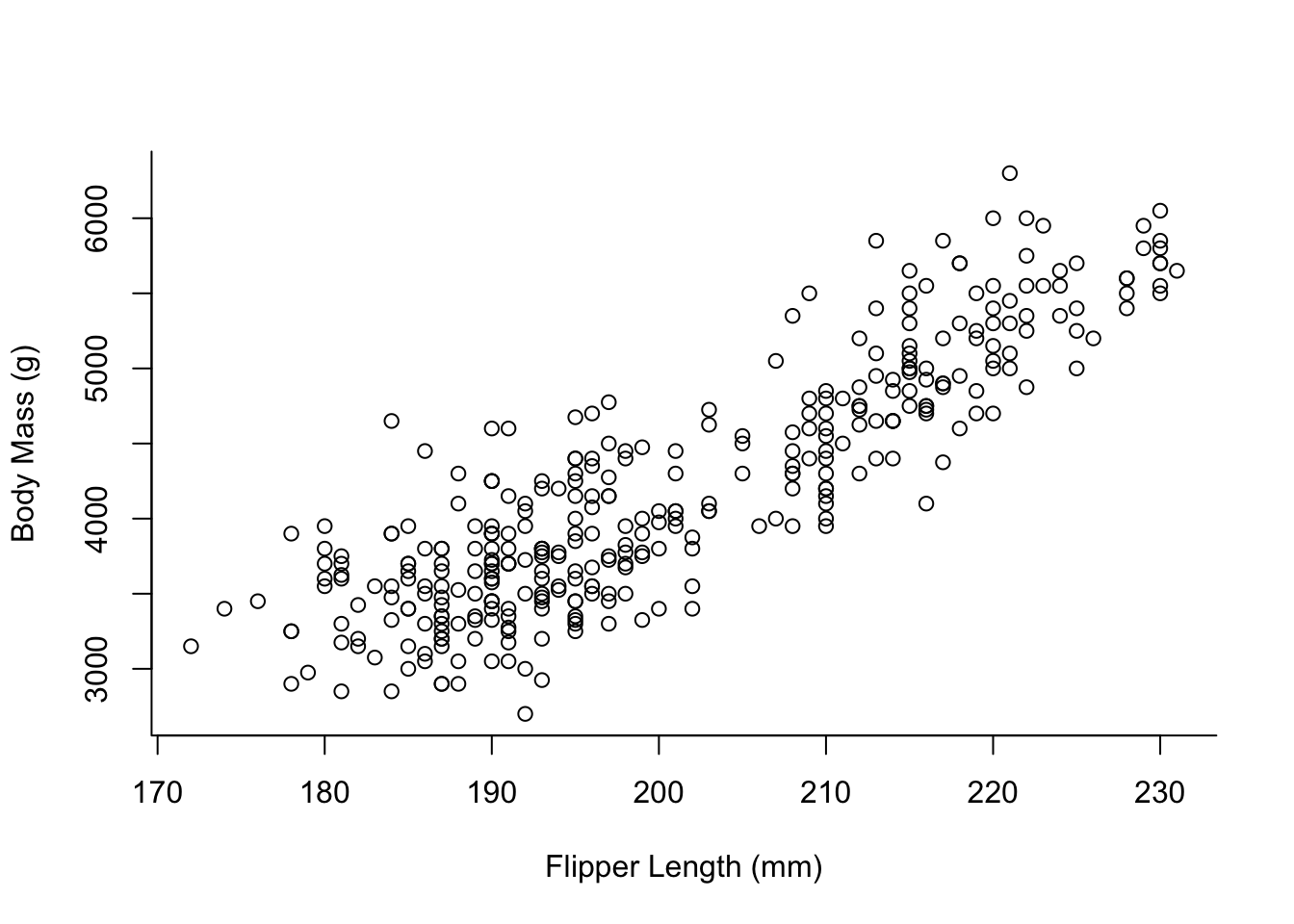The data we use contains information on 3 different species of penguins. For our visualisation we might want to colour them differently.

``````palette(hcl.colors(3, "viridis"))
plot(x    = penguins\$flipper_length_mm,
y    = penguins\$body_mass_g,
col  = penguins\$species,
type = "p",
xlab = "Flipper Length (mm)",
ylab = "Body Mass (g)",
bty  = "l")``````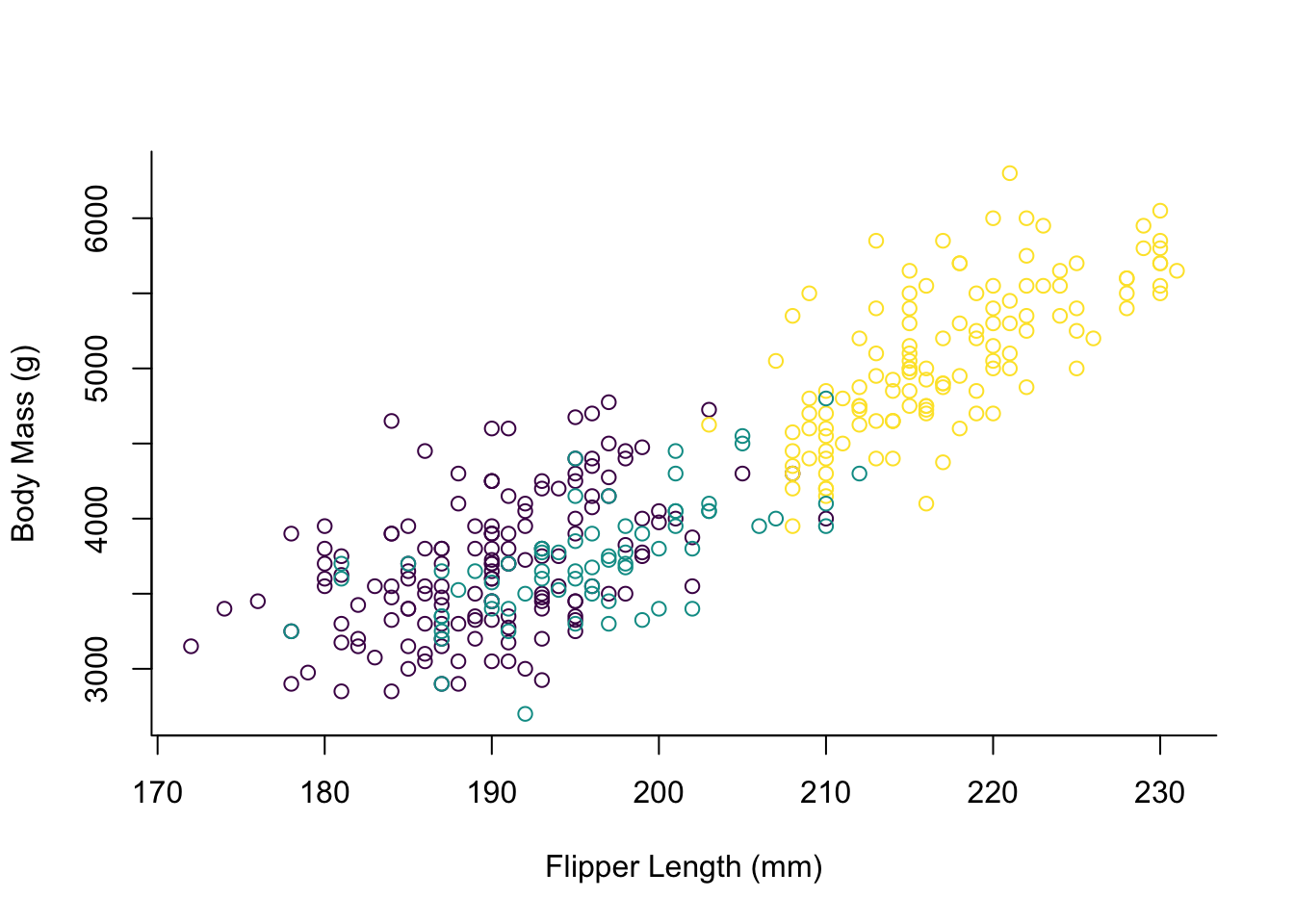The way one has to specify the custom palette here seems to be a bit unusual if you are used to `ggplot2`. By default the points would be coloured red, green and black, but we elect to use the viridis palette here which is colour-blindness friendly.

For some more tweaking the appearance of the plot we should make the points solid and add a legend.

``````par(mai = c(1, 1, 0.4, 1.5))
palette(hcl.colors(3, "viridis"))
plot(x    = penguins\$flipper_length_mm,
y    = penguins\$body_mass_g,
col  = penguins\$species,
pch  = 16,
type = "p",
xlab = "Flipper Length (mm)",
ylab = "Body Mass (g)",
bty  = "l")
legend(x = 235,
y = 6000,
legend = levels(penguins\$species),
col = hcl.colors(3, "viridis"),
pch = 16,
bty = "o",
title = "Species",
xpd = TRUE)``````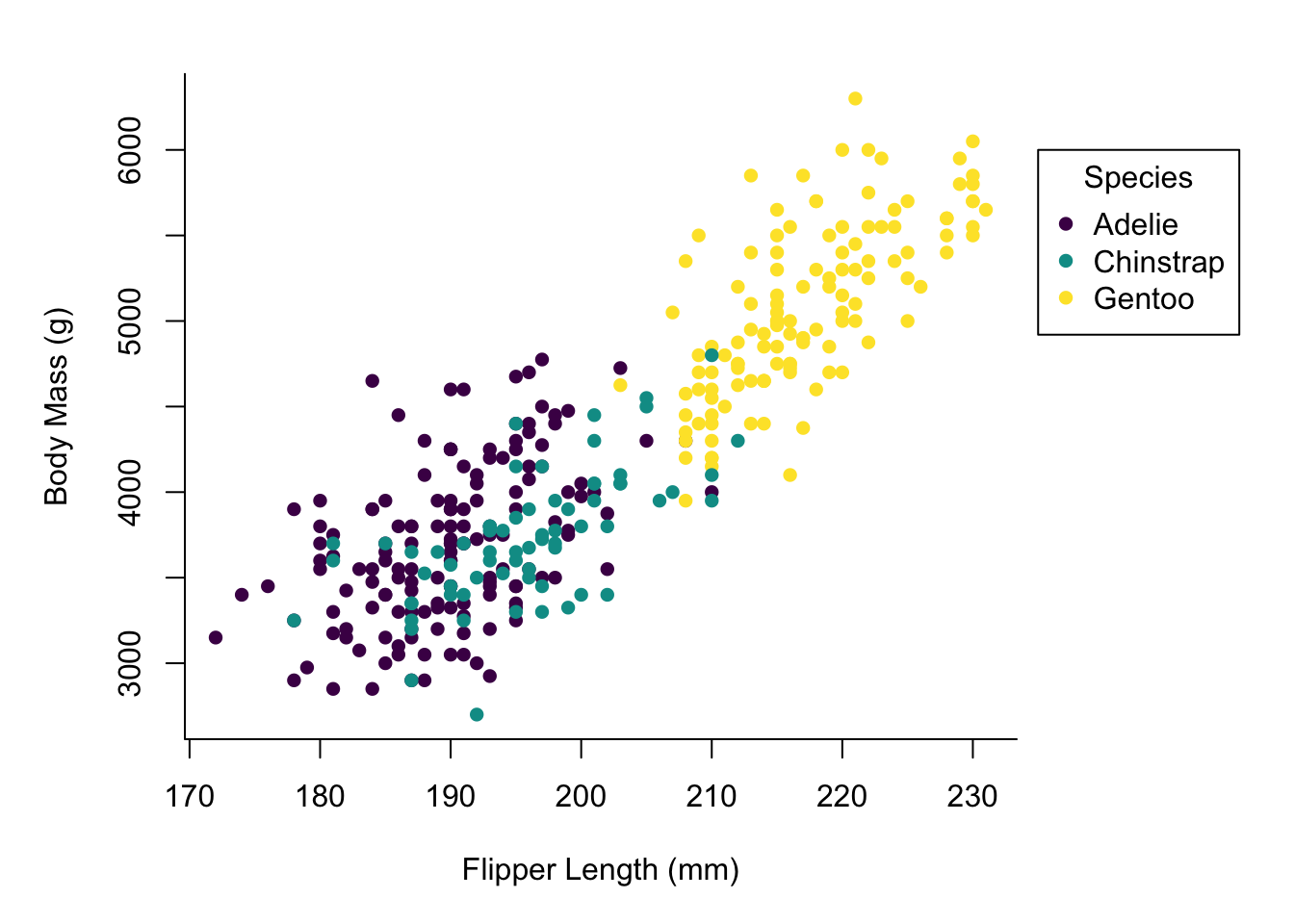Okay, there is a lot of stuff going on here.

One noteworthy thing is that several function calls that appear to be independent act on the same plot. This is very different from `ggplot2` where you chain the things you add to the plot by using `+`. However with base plots plotting functions will act on the currently active plot device until `dev.off()` or `plot.new()` is called.

Here, we first set the margins of our plot, so we can later put our legend outside of the main plotting area. Looking at the documentation for `par`, there are a lot of graphical parameters. A lot of those can be passed to plotting functions (like `bty` in our call to `plot`), but for some more fundamental things it appears to be necessary to set them in a call to `par` before plotting anything.

The call to `plot` now also has the argument `pch = 16` specifying solid points, instead of the circles we had before.

Finally, we have our legend specification. Most of the arguments in there should be straightforward, but note the specification of `xpd = TRUE` allowing us to draw the legend outside of the main plotting area.

At this point we already have a decent looking plot, but we might want to add some finishing touches. First of all, a LOESS smooth line for the relation between flipper length and body mass.

``````mod <- loess(body_mass_g ~ flipper_length_mm, data = penguins)
smooth_data <- predict(mod, penguins, se = TRUE)
idx <- order(penguins\$flipper_length_mm)
smooth_fit <- smooth_data\$fit[idx]
smooth_se_max <- smooth_fit + smooth_data\$se.fit[idx]
smooth_se_min <- smooth_fit - smooth_data\$se.fit[idx]``````

We first need to build the model, then generate the data and retrieve the order of values for properly plotting it.

``````par(mai = c(1, 1, 0.4, 1.5))
palette(hcl.colors(3, "viridis"))
plot(x    = penguins\$flipper_length_mm,
y    = penguins\$body_mass_g,
col  = penguins\$species,
pch  = 16,
type = "p",
xlab = "Flipper Length (mm)",
ylab = "Body Mass (g)",
bty  = "l")
polygon(x = c(rev(penguins\$flipper_length_mm[idx]),
penguins\$flipper_length_mm[idx]),
y = c(rev(smooth_se_max), smooth_se_min),
col = "#00000020",
lty = "blank")
lines(x = penguins\$flipper_length_mm[idx],
y = smooth_se_max,
lty = "dotted")
lines(x = penguins\$flipper_length_mm[idx],
y = smooth_se_min,
lty = "dotted")
lines(x = penguins\$flipper_length_mm[idx],
y = smooth_fit,
col = "black")
legend(x = 235,
y = 6000,
legend = levels(penguins\$species),
col = hcl.colors(3, "viridis"),
pch = 16,
bty = "o",
title = "Species",
xpd = TRUE)``````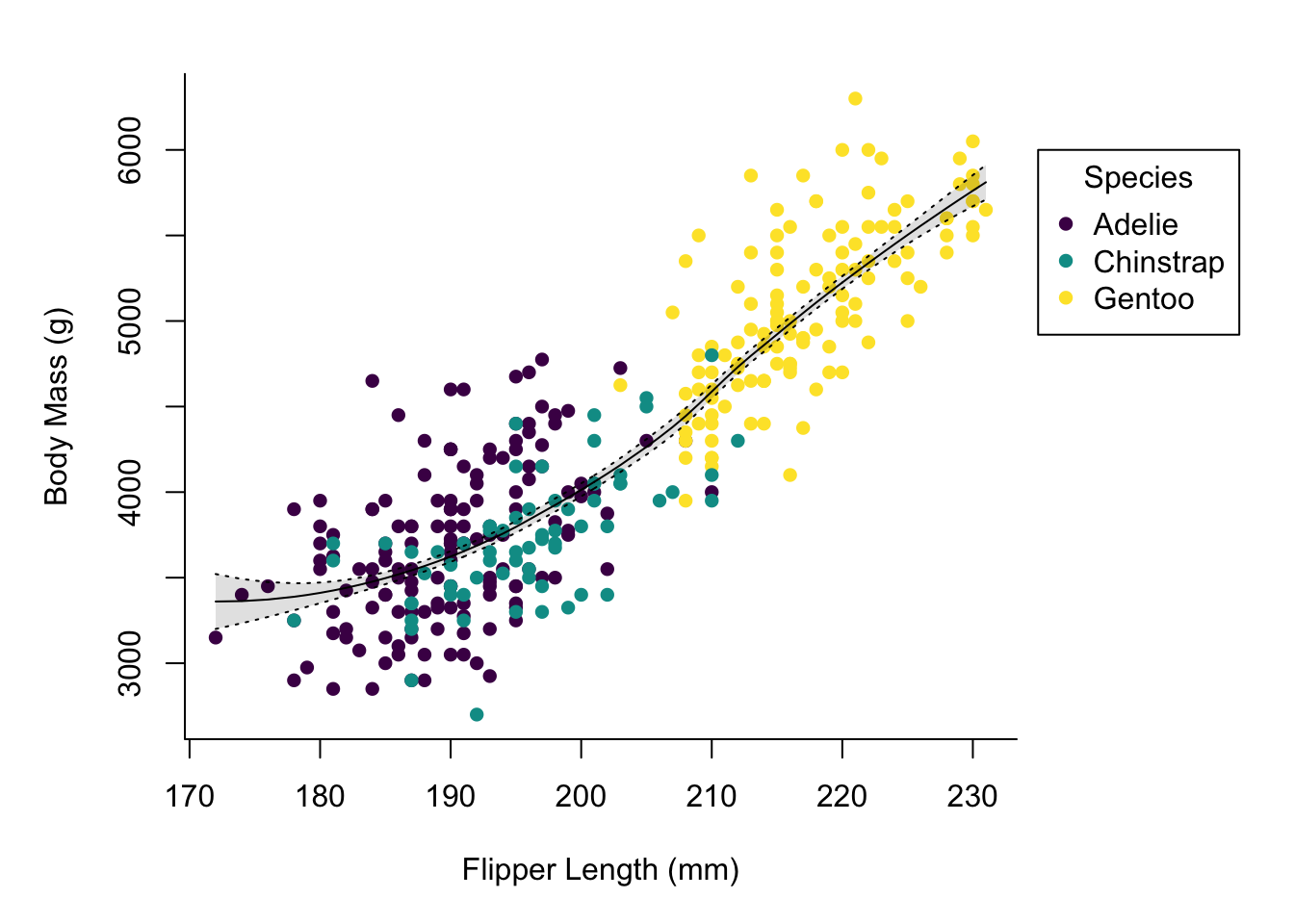In addition to building the model and extracting the relevant values we added a polygon and three more lines to the plot. The polygon is used for the shaded area and I confess, figuring out the right way of building it using `rev` on some of the input data was a bit fiddly.

The additional lines are the LOESS curve on the data and the borders of the region marking the standard error. Using `lty` one can specify a line type, in this case you can just supply a name, which is different from the `pch` argument for points where you must specify a number (but `pch` has much more possible values than `lty`).

Up next we might want to add grid lines.

``````par(mai = c(1, 1, 0.4, 1.5))
palette(hcl.colors(3, "viridis"))
plot(x    = penguins\$flipper_length_mm,
y    = penguins\$body_mass_g,
col  = penguins\$species,
pch  = 16,
type = "p",
xlab = "Flipper Length (mm)",
ylab = "Body Mass (g)",
bty  = "l",
panel.first = grid(lty = "solid", col = "grey90"))
polygon(x = c(rev(penguins\$flipper_length_mm[idx]),
penguins\$flipper_length_mm[idx]),
y = c(rev(smooth_se_max), smooth_se_min),
col = "#00000020",
lty = "blank")
lines(x = penguins\$flipper_length_mm[idx],
y = smooth_se_max,
lty = "dotted")
lines(x = penguins\$flipper_length_mm[idx],
y = smooth_se_min,
lty = "dotted")
lines(x = penguins\$flipper_length_mm[idx],
y = smooth_fit,
col = "black")
legend(x = 235,
y = 6000,
legend = levels(penguins\$species),
col = hcl.colors(3, "viridis"),
pch = 16,
bty = "o",
title = "Species",
xpd = TRUE)``````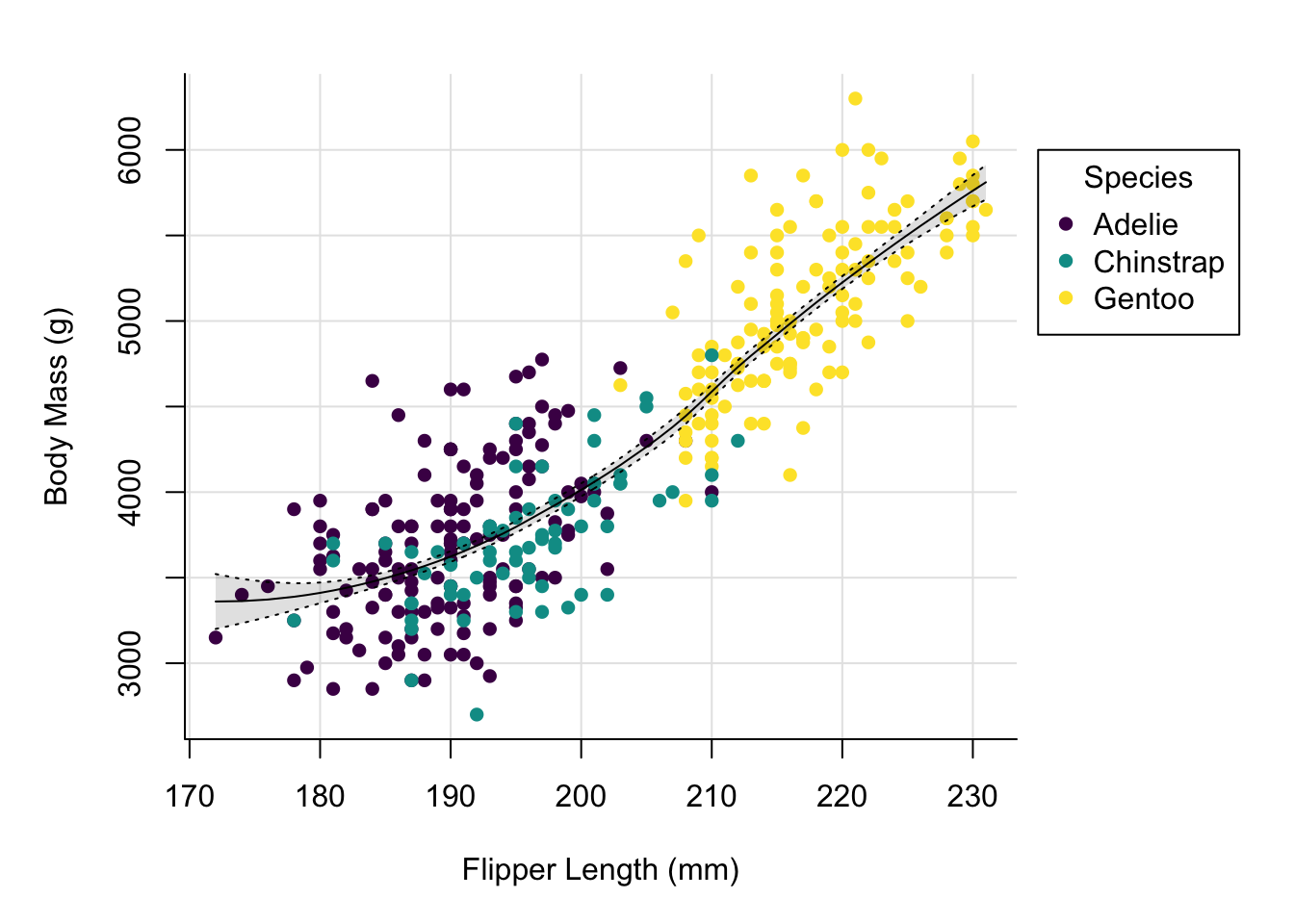By adding the grid using the `panel.first` argument to plot we ensure that the grid is not added on top of our data.

We might want to adjust the x-axis a little, as the break right at the beginning makes the grid line collide a bit with the y-axis.

``````par(mai = c(1, 1, 0.4, 1.5))
palette(hcl.colors(3, "viridis"))
plot(x    = penguins\$flipper_length_mm,
y    = penguins\$body_mass_g,
col  = penguins\$species,
pch  = 16,
type = "p",
xlab = "Flipper Length (mm)",
ylab = "Body Mass (g)",
bty  = "l",
panel.first = grid(lty = "solid", col = "grey90"),
xlim = c(167.5, 230))
polygon(x = c(rev(penguins\$flipper_length_mm[idx]),
penguins\$flipper_length_mm[idx]),
y = c(rev(smooth_se_max), smooth_se_min),
col = "#00000020",
lty = "blank")
lines(x = penguins\$flipper_length_mm[idx],
y = smooth_se_max,
lty = "dotted")
lines(x = penguins\$flipper_length_mm[idx],
y = smooth_se_min,
lty = "dotted")
lines(x = penguins\$flipper_length_mm[idx],
y = smooth_fit,
col = "black")
legend(x = 235,
y = 6000,
legend = levels(penguins\$species),
col = hcl.colors(3, "viridis"),
pch = 16,
bty = "o",
title = "Species",
xpd = TRUE)``````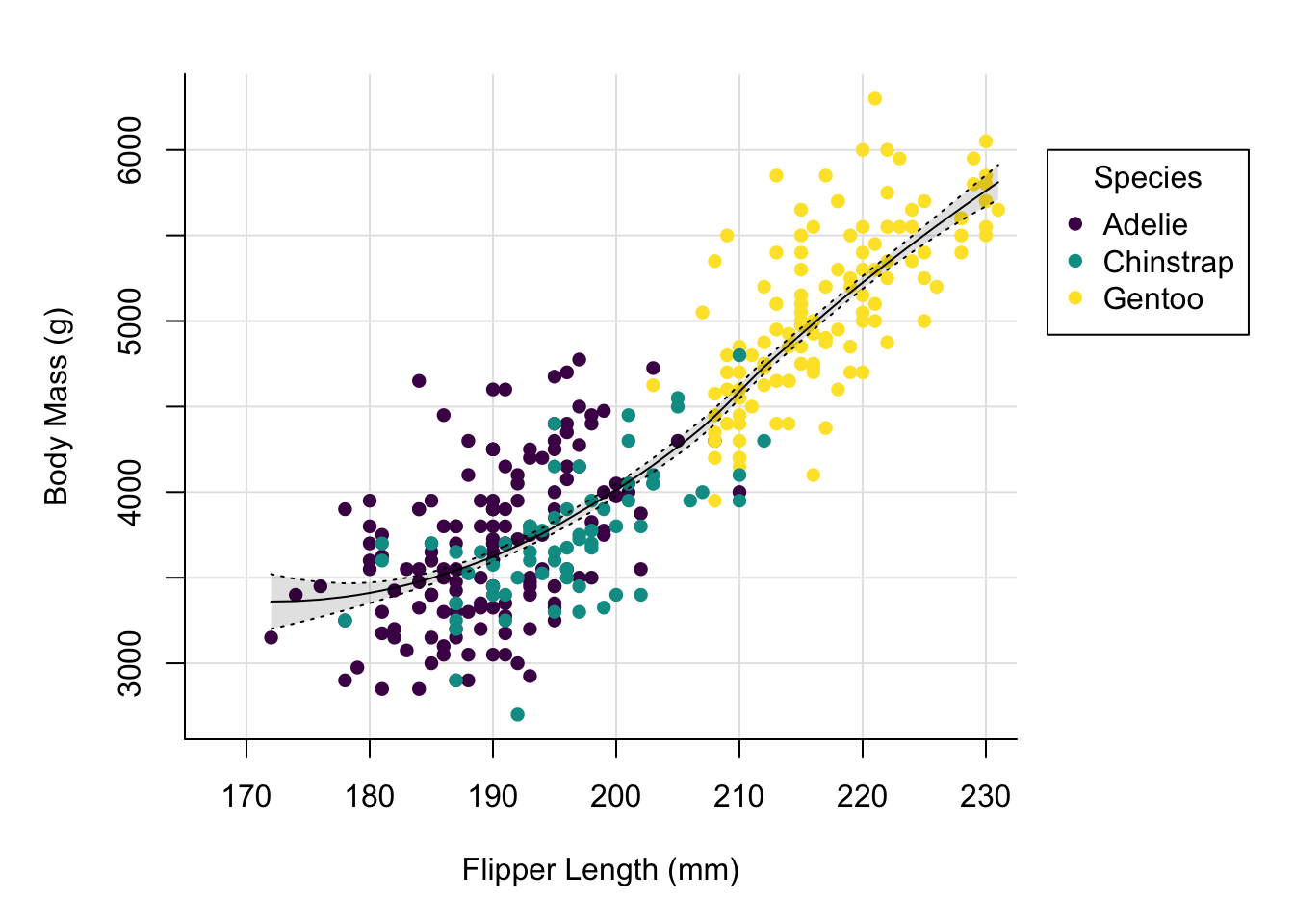This looks nicer. Let’s now add a title and change the font.

``````par(mai = c(1, 1, 0.4, 1.5),
family = "IBM Plex Sans")
palette(hcl.colors(3, "viridis"))
plot(x    = penguins\$flipper_length_mm,
y    = penguins\$body_mass_g,
col  = penguins\$species,
pch  = 16,
type = "p",
xlab = "Flipper Length (mm)",
ylab = "Body Mass (g)",
bty  = "l",
panel.first = grid(lty = "solid", col = "grey90"),
xlim = c(167.5, 230))
title(main = "Size measurements of penguins",
polygon(x = c(rev(penguins\$flipper_length_mm[idx]),
penguins\$flipper_length_mm[idx]),
y = c(rev(smooth_se_max), smooth_se_min),
col = "#00000020",
lty = "blank")
lines(x = penguins\$flipper_length_mm[idx],
y = smooth_se_max,
lty = "dotted")
lines(x = penguins\$flipper_length_mm[idx],
y = smooth_se_min,
lty = "dotted")
lines(x = penguins\$flipper_length_mm[idx],
y = smooth_fit,
col = "black")
legend(x = 235,
y = 6000,
legend = levels(penguins\$species),
col = hcl.colors(3, "viridis"),
pch = 16,
bty = "o",
title = "Species",
xpd = TRUE)``````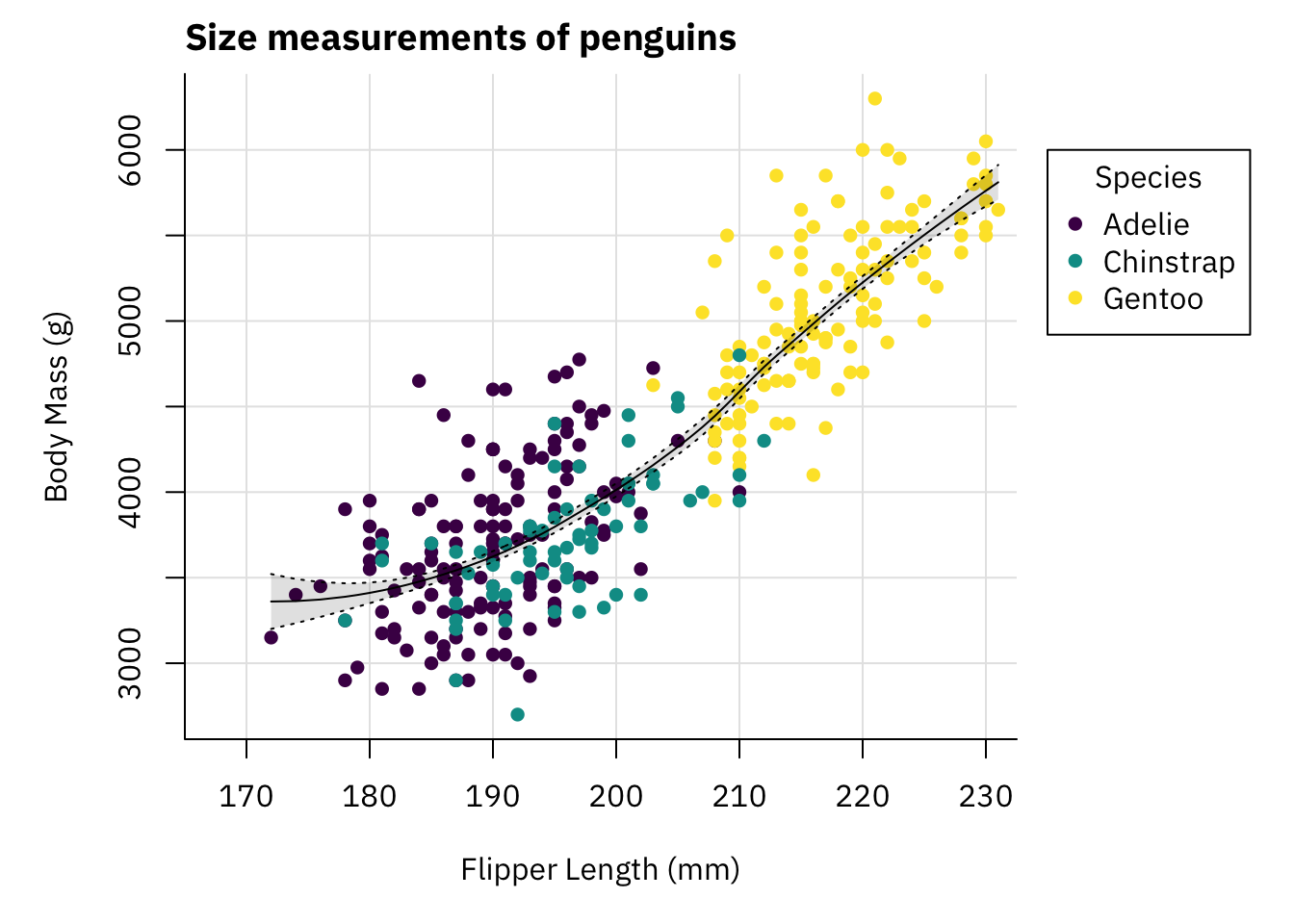This plot looks pretty decent. To be honest if you showed me that plot without context I would not think it was made with base plots.

We can now save it to a file.

``````png("penguins.png", width = 1200, height = 800, res = 150)
par(mai = c(1, 1, 0.4, 1.5),
family = "IBM Plex Sans")
palette(hcl.colors(3, "viridis"))
plot(x    = penguins\$flipper_length_mm,
y    = penguins\$body_mass_g,
col  = penguins\$species,
pch  = 16,
type = "p",
xlab = "Flipper Length (mm)",
ylab = "Body Mass (g)",
bty  = "l",
panel.first = grid(lty = "solid", col = "grey90"),
xlim = c(167.5, 230))
title(main = "Size measurements of penguins",
polygon(x = c(rev(penguins\$flipper_length_mm[idx]),
penguins\$flipper_length_mm[idx]),
y = c(rev(smooth_se_max), smooth_se_min),
col = "#00000020",
lty = "blank")
lines(x = penguins\$flipper_length_mm[idx],
y = smooth_se_max,
lty = "dotted")
lines(x = penguins\$flipper_length_mm[idx],
y = smooth_se_min,
lty = "dotted")
lines(x = penguins\$flipper_length_mm[idx],
y = smooth_fit,
col = "black")
legend(x = 235,
y = 6000,
legend = levels(penguins\$species),
col = hcl.colors(3, "viridis"),
pch = 16,
bty = "o",
title = "Species",
xpd = TRUE)
dev.off()``````

Similar as with `ggplot2` getting sizes right when writing to a file is a bit hard with base plots as well.

So, how do base plots compare to `ggplot2`? One thing `ggplot2` excels at is providing a consistent interface and taking care of a lot of stuff for you based on the aesthetics you specify. For example, here we built the legend manually, `ggplot2` does this automatically for you. Furthermore, `ggplot2` comes with sane defaults and provides plots that at least look okay without much tweaking, while base plots look not so nice out of the box and require some tweaking to look good (this is of course a very subjective thing).

Just from building this example here base plots seem a lot more flexible compared to `ggplot2`. Using `ggplot2` adjusting details can sometimes be kind of hard. With base plots it seems you can just throw some plotting functions at the active graphics device and there are not many limitations. This however comes at the cost of having to repeat yourself a bit, given the plotting functions are independent of each other and are mostly unaware of what has already been plotted.

I have not enough experience with using base plots so I have not run into limitations yet, but from going through the documentation on plotting functions it seems that every little detail of plots can be modified in some way. Thus, using base R plot only you should be capable of producing nice looking and high quality plots.

Nonetheless, after having worked with `ggplot2` for a long time and being fairly happy with the functionality it provides I do not think that I will move to base plots now. While base R plots appear to be capable of producing nice plots, at this point I am so used to using `ggplot2` that it does not seem worthwhile to change my preferred way of plotting. Nonetheless, knowing how to work with base R plots definitely cannot hurt, so I encourage you to at least explore what it can do.